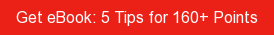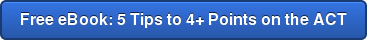# SAT / ACT Prep Online Guides and TipsIn every science class, you must know how to use and interpret the Celsius temperature scale. But this can be difficult for students who are used to using Fahrenheit instead. How do you convert Celsius to Fahrenheit? What about Fahrenheit to Celsius?

We answer these questions below, giving you the mathematical formulas for converting between these two temperature units, a handy conversion chart, and a quick conversion trick you can use without having to grab a calculator.

## Celsius vs Fahrenheit: Key Differences

Before we explain how to convert Celsius to Fahrenheit (and Fahrenheit to Celsius), let’s review the main differences between the two temperature scales.

Celsius (written as °C and also called Centigrade) is the most common temperature scale in the world, used by all but five countries. It’s part of the International System of Units (SI), or what you might know as the metric system, which is typically used in science classes (think centimeters, meters, kilograms, milliliters, etc.) and in science as a whole.

By contrast, Fahrenheit (written as °F) is only used officially by five countries in the world:

• United States
• Belize
• Cayman Islands
• Palau
• Bahamas

Fahrenheit is not part of the metric system; rather, it’s part of the Imperial system, which includes forms of measurements such as inches, feet, pounds, gallons, etc. Moreover, unlike Celsius, it is not typically used in science.

## Celsius to Fahrenheit Formula

Unfortunately, converting from Celsius to Fahrenheit isn’t easy to do quickly or in your head. Here are the formulas used to convert Celsius to Fahrenheit and Fahrenheit to Celsius. These formulas will give you the exact conversion from one unit of temperature to the other:

Celsius to Fahrenheit Formula: (°C * 1.8) + 32 = °F

Fahrenheit to Celsius Formula: (°F - 32) / 1.8 = °C

For example, say the temperature outside is 18 °C and you want to know what this would equal in Fahrenheit. Here’s how your equation would look once you plug in 18 for °C:

(18 * 1.8) + 32
(32.4) + 32
=64.4 °F

Here’s another example if you want to convert Fahrenheit to Celsius: say you’re feeling ill and your body temperature is 101.3 °F. To find out what this equals in Celsius, simply plug 101.3 into the °F part in the second equation written above:

(101.3 - 32) / 1.8
(69.3) / 1.8
=38.5 °C

As you can see, these conversions aren’t particularly hard to carry out, but they do take some time and aren’t the easiest to do without a calculator on hand.

Luckily, there’s a shortcut. By memorizing some of the most common temperatures that come up in daily life, you should have no problem being able to convert from Celsius to Fahrenheit and vice versa. We’ll take a look at how to do this next.It's gettin' hot in here.

## Celsius to Fahrenheit Conversion Chart

Below is a conversion chart listing some of the most commonly used temperatures in everyday life when converting from Celsius to Fahrenheit. Memorize these and you’re sure to have an easier time converting from Celsius to Fahrenheit (and vice versa) fast.

Note: I’ve bolded all temperatures that don’t apply to weather but are still important to know, especially for science class.

 Temperature in Celsius (°C) Temperature in Fahrenheit (°F) Boiling Point of Water 100 212 Extremely Hot Day 40 104 Body Temperature 37 98.6 Hot Day 30 86 Room Temperature 20 68 Chilly Day 10 50 Freezing Point of Water 0 32 Very Cold Day -10 14 Extremely Cold Day -20 -4 Parity* -40 -40

Source: NIST.gov

*The point at which the two temperature units are equivalent (-40 °C = -40 °F).

As you can see from this chart, Fahrenheit temperatures are typically a lot higher than their equivalent Celsius temperatures are.

Also, notice how the difference between Celsius and Fahrenheit starts to get smaller the lower you go in temperature—until, that is, they're the exact same! As the chart indicates, -40 °C is the same temperature as -40 °F. This point is called parity, meaning the two scales use the same value to represent the same temperature. Unfortunately (or fortunately, since this is pretty cold!), you likely won’t come across this temperature in your daily life.

Note that parity only happens at -40°. The lower you go after parity (i.e., the further you go into the negatives), the bigger the difference starts to become again between Celsius and Fahrenheit.

## How to Convert Celsius to Fahrenheit: Quick Trick

If you find yourself needing to quickly convert Celsius to Fahrenheit, here is a simple trick you can use: multiply the temperature in degrees Celsius by 2, and then add 30 to get the (estimated) temperature in degrees Fahrenheit. This rule of thumb is really useful and also pretty accurate for most weather-related temperatures.

For example, if the temperature outside is 15 °C, this would come out to around 60 °F:

(15 * 2) + 30
(30) + 30
= 60 °F (In reality, 15 °C is equivalent to 59 °F—that’s pretty close!)

Of course, expect the actual temperature to be a few degrees off, but for the most part, this is a reliable and easy way to convert temperatures in your head fast.

If you want to convert Fahrenheit to Celsius, do the opposite: subtract 30 from the temperature in degrees Fahrenheit, and then divide by 2 to get the temperature in degrees Celsius.

For example, if the temperature outside is 84 °F, this would be roughly equal to 27 °C:

(84 - 30) / 2
54 / 2
= 27 °C (In reality, 84 °F is equivalent to 28.89 °C—again, that’s a pretty close estimate!).

## What’s Next?

Are you studying clouds in your science class? Get help identifying the different types of clouds with our expert guide.

Now that you know how to convert Celsius to Fahrenheit, the next question to answer is what kinds of spiders live in what sorts of climates? Learn more with our articles about camel spider myths and reasons not to fear the garden spider.

Want even more practice with conversions? (Who wouldn't?) Learn how many cups 4 quarts is here.

Working on a research paper but aren't sure where to start? Then check out our guide, where we've collected tons of high-quality research topics you can use for free.

Need help with English class—specifically with identifying literary devices in texts you read? Then you'll definitely want to take a look at our comprehensive explanation of the most important literary devices and how they're used.

Want to improve your SAT score by 160 points or your ACT score by 4 points? We've written a guide for each test about the top 5 strategies you must be using to have a shot at improving your score. Download it for free now:Hannah Muniz

Hannah received her MA in Japanese Studies from the University of Michigan and holds a bachelor's degree from the University of Southern California. From 2013 to 2015, she taught English in Japan via the JET Program. She is passionate about education, writing, and travel.

Student and Parent Forum

Our new student and parent forum, at ExpertHub.PrepScholar.com, allow you to interact with your peers and the PrepScholar staff. See how other students and parents are navigating high school, college, and the college admissions process. Ask questions; get answers.